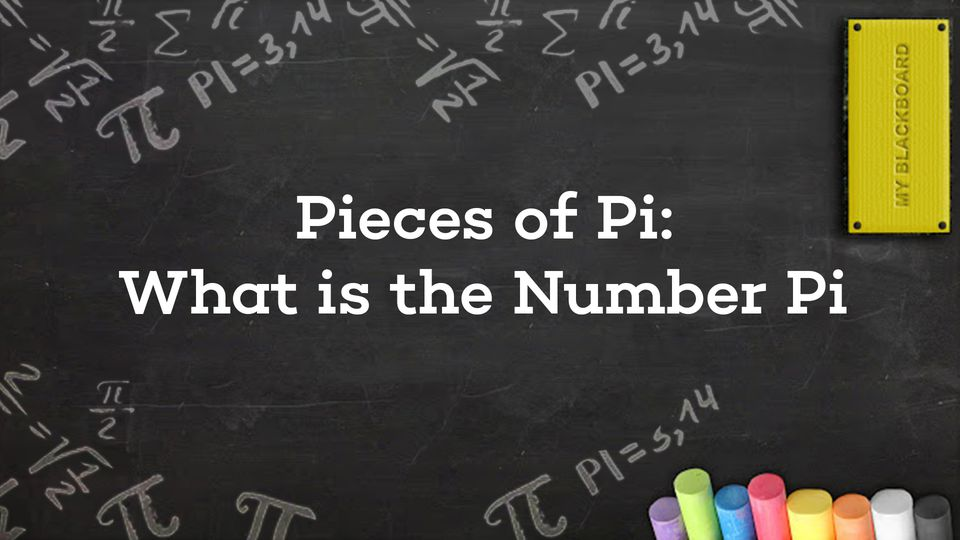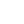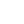Preview the Course
All ages
33m

# Irrational Numbers: What is the Number Pi?

by Robert Abramovic | Science FREE4 Lessons 33:01
1.1. Circles 3:34
2.2. Radius, Center, Diameter, and Circumfernce 8:17
3.3. The Number Pi 2:52
4.4. Examples: Circles Everywhere 18:18

All ages

33m

NGSS Standards

FREE

Course

Circles are everywhere! Tires, wheels, cup lids, flower pots, glass bottoms, coffee pots, signs, and symbols! In this course, you will learn exactly what a circle is as well as three-four important aspects of it: its center, its radius, its diameter, and its circumference. Video examples of circles are given. The irrational number pi is defined as an important ratio: the circumference of a circle divided by its diameter. You will see a claymation video of this division. Two circles are drawn: one from the bottom of a flowerpot and another from the bottom of the glass. You will learn how to measure the circumference and diameter of each circle and see how the number obtained is close to Pi (3.145).

Tags:

• Circles
• Circumference
• Diameter
• Irrational Numbers
• Math
• Pi